# Runtime Polymorphism in C++

## Runtime Polymorphism In C++ With Examples:

In this article, I am going to discuss Runtime Polymorphism in C++ with Examples. Please read our previous article where we discussed Virtual Functions in C++ with Example.

##### Runtime Polymorphism in C++:

This is one of the most important topics in C++ or in object orientation which is Runtime Polymorphism. Runtime polymorphism is also known as dynamic polymorphism or late binding. In runtime polymorphism, the function call is resolved at run time. Now let us see the example of Runtime Polymorphism in C++. So, for that, we will take one class that is Car as shown in the below image.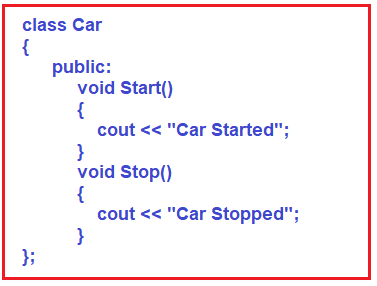This is our Car class. This is which car? This is a generic car or generalized car. For any 4-wheeler which carries passengers, we can say it’s a car. So, the car will be having some functions but here we have defined only Start and Stop functions as public. The Start function will display Car Started and the Stop function will display Car Stopped. Then we have created another class called Innova as shown in the below image.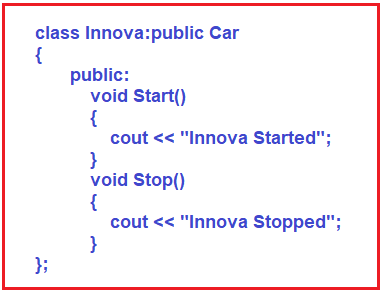The Innova class is inheriting publically from class Car. It means when the Innova class is inheriting from the Car class, then everything the Innova class will get from the Car class. But all those things which are present in Car class are just functions that are Start and Stop. Do they have any role to play? No. They are just displaying a simple message.

We have overridden these functions in the Innova class. That means now both the classes have the same function but in the Innova class, those functions are overridden functions. Now we want to write another class that is Swift class as shown in the below image.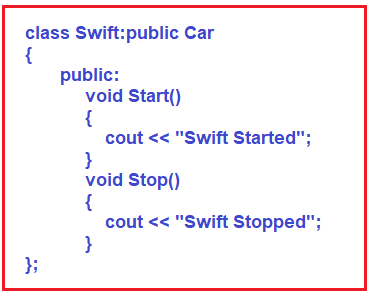The Swift class is also inherited from the Car class. Then inside the Swift class, we have overridden the Start and Stop functions. Already we have taken this example when we were discussing specialization and generalization. So, this example is for generalization. So, do you think which class was existing? Innova was existing and Swift was existing. Then we said that these are cars so we have defined the class car.

Now you can observe one thing here Car class is having two functions. Then what is there with the Car class to share with its children? Who are the child class? Innova is a child class and also Swift is a child class of Car. Is Car class is having anything to share? No.

The Innova and Swift both are overriding the Start and Stop functions. So, what is the use of the Car class? What is the use of those functions when Innova and Swift classes are inheriting from this one? Let us see. Please observe the main function. We have written the following inside the main function.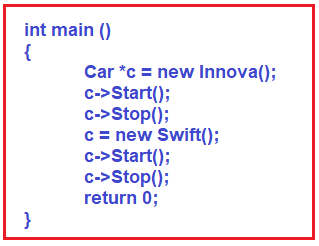Here we have created a pointer c of Car class and first, assigned it to the Innova class object. Then, in the next two lines, with the help of pointer c, we have called Start and Stop functions. Now which function will be called? We have created a base class pointer and assigned it to the derived class object and there are override methods in the derived class. Here, the Car class function will be called. The complete code is given below.

##### Example to Understand Function Overriding in C++
```#include <iostream>
using namespace std;

class Car
{
public:
void Start()
{
cout << "Car Started" << endl;
}
void Stop()
{
cout << "Car Stopped" << endl;
}
};

class Innova:public Car
{
public:
void Start()
{
cout << "Innova Started" << endl;
}
void Stop()
{
cout << "Innova Stopped" << endl;
}
};

class Swift:public Car
{
public:
void Start()
{
cout << "Swift Started" << endl;
}
void Stop()
{
cout << "Swift Stopped" << endl;
}
};

int main()
{
Car *c = new Innova();
c->Start();
c->Stop();
c = new Swift();
c->Start();
c->Stop();
return 0;
}
```
###### Output: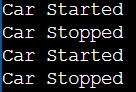Now, suppose we want the derived class function should be called then we need to make the base class functions as virtual functions. This means if we want the Innova class Start function should be called then we have to make the Car class Start function virtual. Similarly, if we want the Innova class Stop function should be called then we have to make the Car class Stop function as virtual. For a better understanding please have a look at the below example.

##### Example to Understand Virtual Functions in C++
```#include <iostream>
using namespace std;

class Car
{
public:
virtual void Start()
{
cout << "Car Started" << endl;
}
virtual void Stop()
{
cout << "Car Stopped" << endl;
}
};

class Innova:public Car
{
public:
void Start()
{
cout << "Innova Started" << endl;
}
void Stop()
{
cout << "Innova Stopped" << endl;
}
};

class Swift:public Car
{
public:
void Start()
{
cout << "Swift Started" << endl;
}
void Stop()
{
cout << "Swift Stopped" << endl;
}
};

int main()
{
Car *c = new Innova();
c->Start();
c->Stop();
c = new Swift();
c->Start();
c->Stop();
return 0;
}
```
###### Output: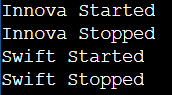So now if we call the c->Start() function then the Innova class Start function will be called. This means that the existence of the Car class is virtual, not real. We use the term Car as virtual. It is a general term. It doesn’t exist in the real world.

Now continuing the main function, in the main function, we assigned the same c pointer to the object of the Swift class. Then on the next two lines, we have called c->Start() and c->Stop() functions. Now, whose function will be called? Now the object is Swift, so Swift’s start and Stop functions will be called. It is like,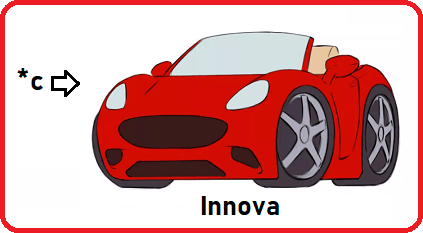First, c was pointing on Innova, so when the Start and Stop function will be called message will be displayed as Innova Started and Innova Stopped.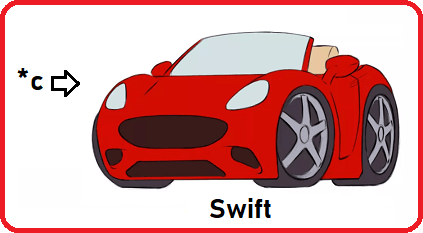Next, when we assigned c to the Swift object then after calling the Start and Stop function, the message will be displayed as Swift Started and Swift Stopped.

Here, the Pointer is the same but the objects are different. So, when we called the function Start depending on the object assigned to it either Swift or Innova class Start function will be called.

As you can see, we execute the same lines that are c->Start() and c->Stop, but the functions called are different. This is Runtime Polymorphism in C++.

We are achieving polymorphism. The same statement but the function calls are different because first the object was Innova and next the object was Swift. It means you can have any car if you have a pointer of the Car assigned to any particular Car object. We can use any car models that has its separate class in the program and that class should be inherited from the Car class. So, the object function will be called which will be assigned to the Car pointer. That’s it. This is an example of Runtime Polymorphism in C++.

Let us extend a few more things here. This is an example of a generalization. Which class is already existing? Swift class and Innova class are already existing. Two classes are already existing. We have written a class Car to achieve polymorphism. Then we have written the common functions in the 3 classes which we have overridden in 2 classes. So why did we write the code in Car class? Is it useful? Are you going to use the object of class Car? No.

So let us remove the code from the Car class and remains the prototype of the functions as follows: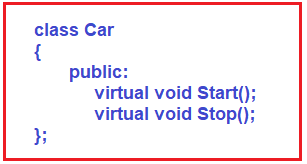Now are these virtual functions? Yes. Do they have the body? No. What is the purpose? That is for just achieving polymorphism. Why do we write function prototypes? Because we want those functions must be implemented by a subclass. So, this Car class became a superclass. We want the functions to be implemented by the subclasses i.e. Innova, Swift, etc.

Have we forced the derived classes to implement these functions? Yes, we want to force. What do we want to force? We want that any class which is inheriting from the Car class then it must override these two functions that are Start and Stop. It must override both functions. How to make it compulsory? We have to equate the function to zero in Car class,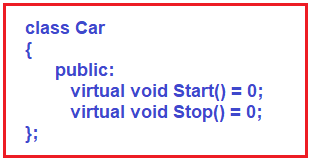So, these functions are known as Pure Virtual Functions. When you assign a virtual function to zero then it becomes a pure virtual function. So, what does it mean or what is the purpose? These functions must be overridden by the derived classes otherwise the derived class will become an abstract class. And the Car class is abstract here.

And, we cannot create the object of an abstract class because it is abstract. When a class inherits from an abstract class then the derived class will also become abstract if that derived class is not overriding the virtual functions. So, we have said that those functions must be overridden. So pure virtual function must be overridden by the derived classes. And the purpose of a pure virtual function is to achieve Runtime Polymorphism in C++.

##### Example to understand Pure Virtual Functions in C++:
```#include <iostream>
using namespace std;

class Car
{
public:
virtual void Start() = 0;
virtual void Stop() = 0;
};

class Innova:public Car
{
public:
void Start()
{
cout << "Innova Started" << endl;
}
void Stop()
{
cout << "Innova Stopped" << endl;
}
};

class Swift:public Car
{
public:
void Start()
{
cout << "Swift Started" << endl;
}
void Stop()
{
cout << "Swift Stopped" << endl;
}
};

int main()
{
Car *c = new Innova();
c->Start();
c->Stop();
c = new Swift();
c->Start();
c->Stop();
return 0;
}
```
###### Output: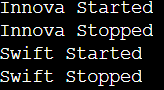You cannot create the object of the Car class. Can we create a reference pointer? Yes, we can have a pointer to the Car class. So, what Car class has to give? Nothing. Just the declaration of the function where the derived classes must implement those functions. So, this is a very interesting and important topic in C++.

##### Key Points of Runtime Polymorphism in C++:
1. Same name different actions
2. Runtime Polymorphism is achieved using function overriding
3. Virtual functions are abstract functions of the base class
4. The derived class must override the virtual functions
5. A base class pointer pointing to a derived class object and an override function is called